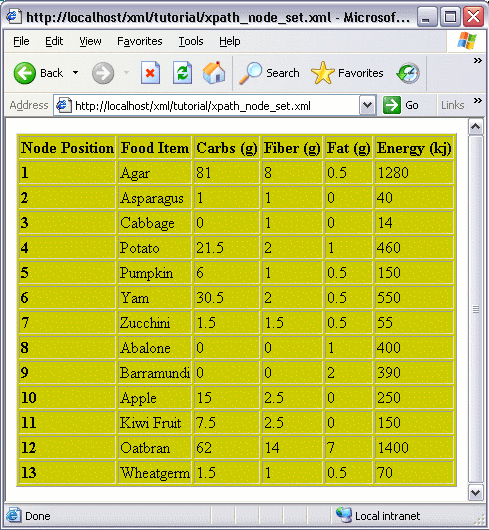# XPath Node Set

A node set is a set of nodes. When you write an XPath expression to return one or more nodes, you call these nodes a node set.

For example, if you use the following expression to return a node called `title`, you will have a set of nodes all called `title` (assuming there's more than one record).

## Node Set Functions

You can use the following functions when working with node sets:

FunctionDescription
last()Returns the number of nodes in a node set.
position()Returns the position of the context node (current node). The starting value is 1. As you loop through each node, the position increments.
count(node1, node2, ...)Returns the total number of nodes in the node set as provided between the parentheses. If you leave the parentheses blank, it will use the context node.
id((string1, string2, ...) node)Returns the nodes whose ID matches the string/s passed to the function.
local-name(node_set)Returns the local name of the first node in the node set. The local name is the name without the namespace prefix. To use the context node, simply leave node_set blank.
namespace-uri(node_set)Returns the URI of the namespace of the first node in the node set. To use the context node, leave node_set blank.
name(node_set)Returns the full, qualified name of the first node in the node set. To use the context node, simply leave node_set blank.

## Node Set Function Example

### The Source XML File

Imagine we have the following XML file:

### The Requirement

Now, imagine we want to display the contents of the file in a table, and that we want to have a `counter` column that provides the count of each row. Something like this:### The Solution

We could achieve the above requirement using the XPath `position()` function, as follows: